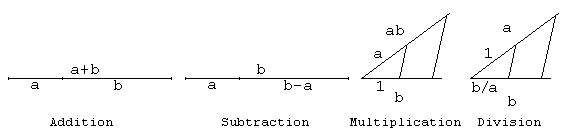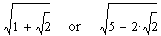# Constructible Numbers

Elsewhere I remarked that introduction of rational numbers had trivialized the operation of division. Division, with its many related concepts (prime, unique factorization, Euclid's algorithm, ...) underlies a whole branch of mathematics, the Number Theory. But, as everyone knows, we still study rational numbers. Rational numbers, of course, have their primordial utility. Which led to their invention in the first place. Dividing whole into parts was as important a task in antiquity as it is nowadays.

For positive m and n, m/n denotes m parts of a unit split into n equal parts. If the whole grows by a certain factor, so do its parts. m/n may equally well stand for the n-th part of the whole that consists of m units. Abstract properties of rational numbers are based on these (everyday) observations. The fundamental one states which fractions are equal:

m1/n1 = m2/n2 iff m1n2 = m2n1,

which abstracts from the fact that increasing the whole by a factor, say p, converts the original n-th part into the (np)-th part of the new total: m/n = (mp)/(np).

We may apply the same ideas to the numbers from Z[m]. So, given two "integers" (a1 + b13) and (a2 + b23) we may consider a "rational" number (a1 + b13)/(a2 + b23). The pleasant thing about this is that such a number proves to be rational in another sense too. For example,

(a1 + b13)/(a2 + b23) = r + q3,

where r = (a1a2 - 3b1b2)/N(a2 + b23) and q = (a2b1 - a1b2)/N(a2 + b23) are both rational numbers in the ordinary sense (i.e. belonging to Q.) This is how the terminology comes about. Let the set of all numbers a + bm (m is not a complete square) be denoted Q[m]. The numbers from Z[m] are often just called integers, whereas the numbers from Q[m] are rational in the same context. Q[m] is closed under all four arithmetic operations: addition, subtraction, multiplication, and division. Which makes Q[m] a field. This is the extension (or Galois) field of Q by m. Extension fields underlie the theory of geometric construction.### Geometric Constructions

The notion that geometric constructions should be performed with a straightedge and a compass predates Euclid's Elements. Archimedes' approach to the famous Angle Trisection problem furnished a practical way of dividing an angle into three equal parts but was not (and is not) considered as solving the problem because its dependency on the device of having a segment of constant length slide along a moving straight line. It is impossible to implement this device with a straightedge and a compass. This so happens that the Angle Trisection problem can't be solved in general (i.e., for an arbitrary angle) if the straightedge and compass are the only two instruments allowed. Analytic Geometry and Extension fields combine to clarify the picture.The four basic arithmetic operations are easily seen to be implementable with elementary geometric constructions. The diagram outlines the ways to find a+b, a-b, ab, and a/b for any two positive numbers. For the latter two, we also need a segment of unit length. Given such unit segment, we may first construct segments whose length is expressed as an integer, and then as a rational number.The same set of similar triangles that helped prove the Pythagorean Theorem shows how to construct a square root of a number. If we call numbers that express lengths of segments constructed with a straightedge and a compass constructible, then we find that all (positive) integers and rational numbers are constructible as are the square roots of such numbers.

This shows that starting with a unit interval and using a straightedge and a compass we are able to construct any number that belongs to any of the fields Q[m]. We may go further and use one of Q[m], say Q, instead of Q. For various m, then, we shall have fields Q[m] that in a little more concise form are written as Q[2,m]. For example, Q[2,3] of numbers in the form a + b3 where a and b belong to Q.

Note that 3 ∈ Q while 3 does not. To extend this field we may have chosen any of its elements whose root does not belong to the field.may have been used to extend Q. The process may continue with the new field. So that in general after N steps we would have a field denoted as Q[m1,m2,...,mN], where numbers mi are such that none of them is a complete square in the extension field Q[m1,m2,...,mi-1]. Any number from any of the fields Q[m1,m2,...,mN] is constructible.

Conversely, any constructible number belongs to one of the extension fields Q[m1,m2,...,mN]. To see this, assume that a field F contains only constructible numbers, and imagine a Cartesian plane where all our constructions take place. According to the assumption, with a straightedge and a compass we know how to depict points with coordinates from F. Straight lines drawn through such points have coefficients that belong to F. A point of intersection of two such lines has coordinates that still belong to F. Using a circle brings some variety: To find a point of intersection of a straight line and a circle or points of intersection of two circles, we have to solve a quadratic equation. If the roots do not belong to F, we may use it to extend the field F. To sum up, let there be a constructible number a. This simply means that the number was determined through a sequence of geometric constructions with a straightedge and a compass. The sequence is finite, and at every step we did something simple: drew a line or a circle or found the intersection of the two lines drawn previously. On the first step, all possible elements either had coordinates (points) or coefficients (lines) that belong to Q. This state of affairs lasts until we attempt to determine points of intersection with a circle. Sometimes the resulting points will have coordinates in F = Q. If not, we'll have to replace Q with an extension field F = Q[m1] for some m1 which is not a complete square in Q. For a few steps we may stay in the framework of F = Q[m1]. But if the construction requires us again to find points of intersection with a circle, we may have to extend the field further and start working with F = Q[m1,m2]. The fields, if necessary, extend until the number α crops up in the course of the construction.Every constructible number is algebraic. In other words, every constructible number α is a root of a polynomial equation with integer coefficients

Pn(x) = anxn + an-1xn-1 + ... + a1x + a0 = 0

The proof us by induction on the number N of extension fields needed to include α into Q[m1, m2,..., mN].

For m = 0 there is nothing to prove. Any rational number m/n solves a polynomial equation of degree 1: nx - m = 0. Assume that the assertion has been proven for any α ∈ F = Q[m1,m2,...,mK]. Let then α ∈ F[m] where m is not in F but m is. α = a + bm with a, b, and m all elements of F. If b = 0, then α ∈ F and, by the inductive assumption, it is algebraic. Otherwise, m = (α - a)² / b² ∈ F and is, therefore, a root of an algebraic equation:

P((α - a)² / b²) = 0.

The degree of this equation is double that of the polynomial P; its coefficients all belong to F = Q[m1,m2,...,mK]. The latter means that each of the coefficients is representable in the form c + dmK with c and d belong to Q[m1,m2,...,mK-1]. This means the equation may be rewritten as, say Q(α) + R(α)mK = 0, or Q(α) = -R(α)mK, squaring which (and, thus, doubling again the degree of the equation) we'll get a polynomial equation for α with coefficients from the field Q[m1,m2,...,mK-1]. Continuing this process we shall eventually come up with a polynomial equation with rational coefficients satisfied by α. Multiply the polynomial by the product (or any common multiple) of the denominators of its coefficients to get an equation with integer coefficients. This proves α is algebraic.

Since the set of all algebraic numbers is countable, we get, as an immediate consequence of this result, that the set of all constructible numbers is also countable. Not all algebraic numbers are constructible. For example, the roots of a simple third degree polynomial equation x³ - 2 = 0 are not constructible. (It was proved by Gauss that to be constructible an algebraic number needs to be a root of an integer polynomial of degree which is a power of 2 and no less.) As we shall see later on, this gives a negative solution to the problem of Doubling the Cube, one of the three famous problems of antiquity.

### References

1. R. Courant and H. Robbins, What is Mathematics?, Oxford University Press, NY, 1996.
2. H. Dorrie, 100 Great Problems Of Elementary Mathematics, Dover Publications, NY, 1965
3. M. Lascovitch, Conjecture and Proof, MAA, 2001
4. I. Stewart, Concepts of Modern Mathematics, Dover, 1995### Constructible Numbers, Geometric Construction, Gauss' and Galois' Theories